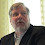## Monday, December 23, 2013

### Dimensional Analysis is an Important Modeling Tool

Modeling is about units and keeping the units straight is critical.   Dimensional analysis assures that we are comparing “apples to apples” and that we are in the correct ball park with our answers.  Most of you probably already know this but some of you perhaps do not.

I once reported an answer that should have been in units of milligrams (mg) as micrograms (µg) and thus released a report with an error of 1000x !   That mistake provided two lessons.   First, ALWAYS have some peer review by a trusted colleague and second, take your time and do a thorough dimensional analysis of your math.

Most exposure models represent a string of algebraic calculations.   Sometime the string can get pretty long and complicated with all the factors that go into making the model prediction on the left hand side of the equation and the answer (either final or intermediate answer) on the right side of the equal sign.  If you break it down using dimensional analysis, it becomes much easier to handle.

Let’s do an example of a relatively simple equilibrium concentration model with constant source rate and ventilation rate:   C = G/Q     Note:  we want our answer in mg/ m3

The scenario is an open drum evaporating into a room at room temperature.

For Q we are told that it is a 30 m3 room with 0.5 mixing air changes of fresh air ventilation per hour.
So  Q = (room vol)(air changes per hour) =  units of  (m3)(1/hr) or m3/hr  ans:  15 m3/hr

Simple enough, indeed I think we have all done this; however, look closely at what we really did.  We multiplied a variable with units (m3) times a variable with units  (1/hr or hr-1) to get an entity with units m3/hr.   That is really all there is to dimensional analysis.

Let’s say we measured the evaporative loss of liquid from the drum over time as 2 grams in 400 minutes.   That is 2/400 or 0.005 grams/min; however, we are looking for units of mg/hr.     So:

G = (0.005 grams/min)(60 min/hr)(1000 mg/gram) =   300 mg/hr   (we cancel out the grams and the minutes and are left with mg/hr

As such,  we have C = to G (300 mg/hr) divided by Q (15 m3/hr).   For clarity I am just showing just the units or dimensions below:
(mg/hr)/(m3/hr) and the hrs cancel out leaving mg/m3

In the above equation we are left with 20 mg/m3 as an estimated equilibrium airborne concentration in this room.

If we knew the molecular weight of the compound we could calculate the concentration as volume parts per million (ppm v/v) in air using the molar volume (gaseous volume of 1 mole of any gas or vapor) of 24 liters (L) at 25C.   For a vapor at 1 mg/m3 with a MW of 100 g/mole we can determine the linear conversion factor:

(1g/1000mg)  (24L/mole) (1 mole/100 g) (0.001 m3/L)(1 mg/m3)= 2.4 x 10-7 (unitless conversion factor)

Thus, for every 1 mg/m3 of a gas (with MW 100 at 25C) there are 2.4 x 10-7 parts of gas per volume parts of atmosphere.  Multiply this part per part by one million (106) and you have the parts per million conversion factor of 0.24 for the conversion between mg/m3 and ppm v/v or 1/0.24 =  4.2 to convert ppm v/v to mg/m3 for this gas at this temperature.  The dimensional analysis for this is below:

For every 1 mg/m3 there are (2.4 x 10-7 parts/parts) (1,000,000 parts/million parts by volume) =  0.24 ppm v/v and the receprical 1/0.24 or 4.2 mg/m3 for every ppm v/v of this vapor.  For our example it would be 20 mg/mtimes 0.24 or 4.8 ppm v/v assuming its MW was 100 and it was at 25C.

If this explanation of dimensional analysis is a little fuzzy, I found one that is clearer and is only 9 minutes long on YouTube:    http://www.youtube.com/watch?v=fEUaQdaOBKo

Believe me, dimensional analysis is your friend and will help to keep you sane in doing problems associated with modeling.

1.1.